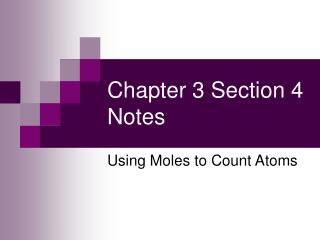# Chapter 3 Section 4 Notes - PowerPoint PPT PresentationDownload PresentationChapter 3 Section 4 Notes

Chapter 3 Section 4 NotesDownload Presentation## Chapter 3 Section 4 Notes

- - - - - - - - - - - - - - - - - - - - - - - - - - - E N D - - - - - - - - - - - - - - - - - - - - - - - - - - -
##### Presentation Transcript

1. Chapter 3 Section 4 Notes Using Moles to Count Atoms

2. What is a mole? • The SI base unit that describes the amount of a substance; a collection of a very large number of particles.

3. What is a mole useful for? • Counting small particles

4. Avogadro’s Constant • Avogadro’s Constant: the number of particles in 1 mol • Equals 6.022 x 1023 particles/ mol • 602,213,670,000,000,000,000,000 particles • How does Avogadro’s constant relate to a mole of something? • It is the number of particles in one mole of anything.

5. Moles and Grams are related • Molar Mass: the mass in grams of 1 mol of a substance • How do you find molar mass? • The average atomic mass is equal to molar mass. • Simply look on the Periodic Table!

6. Practice • Determine the molar mass of the following elements: (Round to the nearest hundredth) • Manganese, Mn • 54.94 g/mol • Cadmium, Cd • 112.41 g/mol • Arsenic, As • 74.92 g/mol • Strontium, Sr • 87.62 g/mol

7. More Practice • Determine the mass, in grams of each of the following: • 0.48 mol of platinum • 2.50 mol of sulfur 0.48 mol x 195.08 g = 94 g 1 mol 2.50 mol x 32.07 g = 80.2 g 1 mol

8. Even More Practice • Determine how many moles are present in each of the following: • 620 g of mercury • 11 g of silicon 620 g x 1 mol = 3.09 mol 200.59 g 11 g x 1 mol = 0.39 mol 28.09 g

9. Compounds also have molar mass • To find the molar mass of a compound, add up the molar masses of all the atoms in a molecule of the compound. • Example: H2O • Molar Mass of Hydrogen: 1.01 x 2 = 2.02 g/mol • Molar Mass of Oxygen: 16 g/mol • Total Mass of H2O: 2.02 g + 16 g = 18.02 g/mol